# 天津小学二年级加减乘除法混合运算练习题天津小学二年级加减乘除法混合运算练习题

7×8=      9×4=      8×8=      7×8=

7+8=      9+4=      8+8=      7+8=

25+4×6=       9×9-30=       2×8+44=

6×9-45=       50-9×4=       7×7+11=

54+3×2=       6×9-9=        8×9+9=

54÷6=     12÷6=     12÷2=     72÷9=      36÷9=

12÷3=     14÷2=     30÷5=     54÷9=      10÷5=

65÷7=     26÷3=     17÷8=     32÷4=      78÷9=

54÷9+15=    63÷7+35=      42-48÷6=

32÷4-5=     21+18÷6=      81÷9+53=

3×2×6=      49÷7×4=      54÷9×4+16=您还可以通过手机、平板电脑等移动设备访问天津奥数网，升学路上有我们相伴。>>[点击查看]

•欢迎扫描二维码
关注奥数网微信
ID：aoshu_2003

•欢迎扫描二维码
关注中考网微信
ID：zhongkao_com

### 小学新闻导航

1.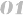北京小学新闻 上海小学新闻 广州小学新闻 深圳小学新闻
2.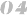天津小学新闻 武汉小学新闻 成都小学新闻 石家庄小学新闻
3.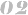南京小学新闻 杭州小学新闻 济南小学新闻 苏州小学新闻
4.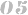郑州小学新闻 沈阳小学新闻 太原小学新闻 重庆小学新闻
5.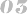长沙小学新闻 合肥小学新闻 宁波小学新闻 青岛小学新闻